Lab 03 Parameter Analyzer

Hung, Bill Chun Wai

Objectives

To familiarize the use of the Agilent parameter Analyzer, and to understand the linear and non-linear devices.

Procedure/Measurement Report

1. Perform analysis of the Circuit 1a, Circuit 1b, Circuit 2a, Circuit 2b with the Agilent parameter Analyzer.

2. Perform analysis on a diode in a chip with the Agilent parameter Analyzer.

Results/Analysis

Circuit 1a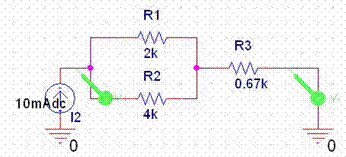PSpice Plot 1a.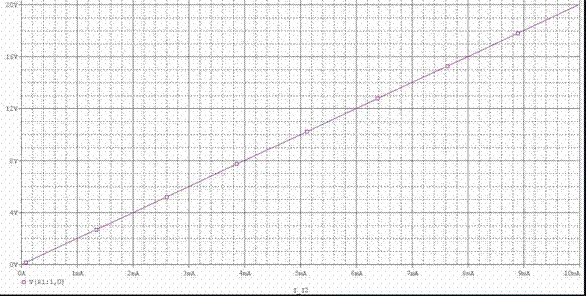Calculation: The equivalent resistance of the circuit is 2k//4k + 0.67k = 2k. The plot of the I-V plot should be a straight line. When the current through the circuit is 0mA, the voltage should be 0V. When the current through the circuit is 10mA, the voltage should be V=IR=10mA x 2k = 20V.

The PSpice plot is consistence with the calculated value (20V), and the experimental plot (next page) is also consistence with the calculated value(20V).

Part 1b.

Circuit 1b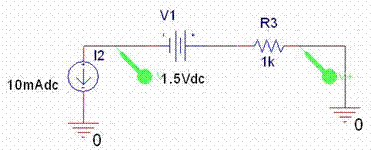PSpice Plot 1bCalculation: The DC voltage source gives an offset of 1.5V to the circuit. Besides the voltage source, there is only one resistor. So the plot is a straight line. When the current is 0A, the voltage is 0V + 1.5V = 1.5V because of the offset. When the current is 10mA, the voltage is V=IR + 1.5V=10mA x 1k + 1.5V= 11.5V.

The calculated value (11.5V) agrees with the PSpice plot shown above. The experimental result also generates the same result, and the plot of the experimental result is shown in the next page. The experimental result also agrees with the calculated value (11.5V).
Part 2a.

Circuit 2a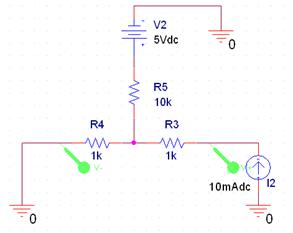PSpice Plot 2a1

Calculation: the V2 (Vg) gives an offset to the circuit. When the current is 0mA, and the V2 (Vg) is 0V, the voltage across R4 and R3 (Vds) is 0V. When the current is 10mA, and the V2 (Vg) is 0V, the voltage across and R3 (Vds) is V=IR = 10mA(1k + 10k//1k) = 19.1V.

The V2 (Vg) is 1V, the V2 (Vg) gives and offset of 1k/(1k+10k) = 0.09V. That¡¯s the I-V straight line curve when V2(Vg) is 0V shifted upward by 0.09V. When the V2 (Vg) is increased by another 1V (2V total), the output voltage curve is shifted by 2 x 0.09V = 0.18V. That¡¯s 1V increase in V2(Vg) results in an offset of 0.09V of the output voltage. The voltage of V2 (Vg) of 0V to 5V is shown in the Plot.

The calculation agrees with the PSpice plot. However, there was an error while the experiment was conducted, and the experimental plot is shifted upward more than predicted.

Part 2b

Circuit 2b Open Switch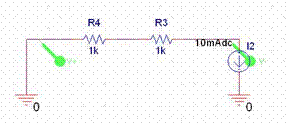PSpice Plot 2b Open SwitchCircuit 2b Close Switch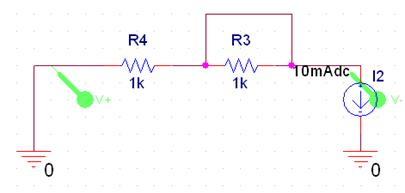PSpice Plot 2b Close Switch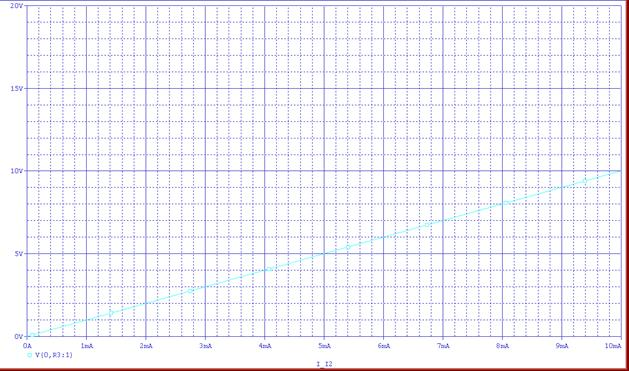When the switch is open, the equivalent resistance is 2k, and when the current through the resistors is 10mA, the voltage is V=IR = 20V. This calculated results agrees with the PSpice Plot and the experimental I-V plot.

When the switch is closed,  one the of 1k resistors is shorted out. The resulted circuit is the closed circuit with only 1k equivalent resistance. When the current is 10mA, the voltage is V=IR = 10K. This calculation agrees with the PSpice plot as well as the experimental plot shown on the next page.

Part 3. Diode

When the Voltage across the forward-biased diode is below the cutoff voltage, the diode is off. In other words, the current through the diode is very low. Once the voltage across the diode reaches the cutoff voltage, the diode acts as a virtual short circuit and permits current to go through.

The ideal plot of the I-V curve of a forward biased diode should be a step function, however, the experimental plot obtained from the lab is not a perfect step function. This is because the diode used in the lab is not a perfect diode.

Conclusions

Through this lab, the use of the parameter analyzer is explored, and familiar circuits of resistors, diodes are plotted. This gives us a general sense how to use the parameter analyzer to perform analysis. This lab also introduce the use of PSpice different circuits.